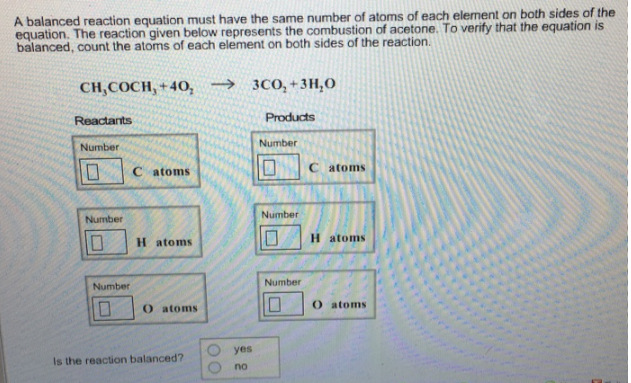# Problem: A balance reaction equation must have the same number of atoms of each element on both sides of the equation. The reaction given below represents the combustion of acetone. To verity that the equation is balanced, count the atoms of each element on both sides of the reaction. CH3COCH3 + 4O2 → 3CO2 + 3H2O Is the reaction balanced?                   (i) yes                   (ii) no

###### FREE Expert Solution
100% (308 ratings)###### Problem Details

A balance reaction equation must have the same number of atoms of each element on both sides of the equation. The reaction given below represents the combustion of acetone. To verity that the equation is balanced, count the atoms of each element on both sides of the reaction.

CH3COCH3 + 4O2 → 3CO2 + 3H2

Is the reaction balanced?

(i) yes

(ii) no